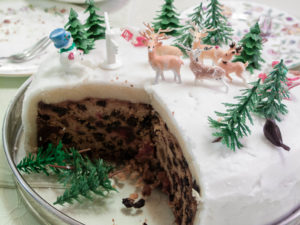## Putting It Together: Fractions

At the beginning of this module, we met Noelle who was planning her holiday baking. She wants to make as many cakes as she can with the flour she has on hand and with what is left, she would like to make cookies. Noelle has 13$\frac{1}{2}$ cups of flour, and her cake recipe calls for 2 cups of flour, and can make 6 cookies per $\frac{1}{2}$ cup of flour.First, Noelle determines how many cakes she can make by dividing the total amount of flour she has by 2. Since it doesn’t make sense to make a fraction of a cake, she will set aside any portion that is less than 2 cups.

In the module, we fist converted mixed numbers to improper fractions, then divided. We will do that to determine how many cakes (2 cups of flour each) we can make with $13\frac{1}{2}$ cups of flour.

$13\frac{1}{2}\div 2 = \frac{27}{2}\cdot\frac{1}{4} = \frac{27}{4} = 6\frac{3}{4}$

We can interpret this as $6\frac{3}{4}$ cakes can come out of $13\frac{1}{2}$ cups of flour. Since we don’t want $\frac{3}{4}$ of a cake, we will just use enough flour to make 6 cakes.

$6\cdot 2 = 12$ cups of flour are needed to make 6 cakes.

If we started with $13\frac{1}{2}$ cups of flour and use $12$ we will have $1\frac{1}{2}$ cups left for making cookies.  We can now determine how many cookies Noelle can make.

For each $\frac{1}{2}$ cup of flour, she will get $6$ cookies, if $1\frac{1}{2} = \frac{3}{2}$, this means Noelle has $3$ half cups of flour, so can make $3\cdot6 = 18$ cookies.

Noelle has enough flour to make 6 cakes, and 18 cookies with the flour she has on hand.

## Contribute!

Did you have an idea for improving this content? We’d love your input.